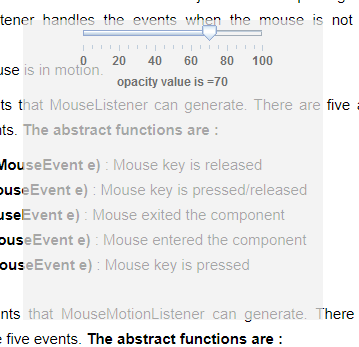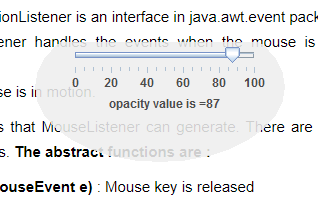# Java Swing | Translucent and shaped Window in Java

Java provides different functions by which we can control the translucency of the window or the frame. To control the opacity of the frame the frame must not be decorated. Opacity of a frame is the measure of the translucency of the frame or component.
In Java, we can create shaped windows by two ways first by using the AWTUtilities which is a part of com.sum.awt package AWTUtilities class have a function – setWindowShape(Window w, Shape s) which sets the shape of the window and second by using setShape(Shape s) which sets the shape of the window to the specified shape.

Methods used :

1. setWindowShape(Window w, Shape s) : sets the shape of the window w to the specified shape s
2. setShape(Shape s) : sets the shape of the window to the specified shape s
3. setOpacity(float f) : sets the value of opacity of the frame

1. Program to create a translucent frame and control its translucency with the help of a JSlider

 `// Java  program to create a ` `// translucent frame and control ` `// its translucency with ` `// the help of a JSlider ` `import` `javax.swing.event.*; ` `import` `java.awt.*; ` `import` `javax.swing.*; ` `class` `solveit ``extends` `JFrame ``implements` `ChangeListener { ` ` `  `    ``// frame ` `    ``static` `JFrame f; ` ` `  `    ``// slider ` `    ``static` `JSlider b; ` ` `  `    ``// label ` `    ``static` `JLabel l; ` ` `  `    ``// main class ` `    ``public` `static` `void` `main(String[] args) ` `    ``{ ` `        ``// create a new frame ` `        ``f = ``new` `JFrame(``"translucent window"``); ` ` `  `        ``// create a object ` `        ``solveit s = ``new` `solveit(); ` ` `  `        ``// create label ` `        ``l = ``new` `JLabel(); ` ` `  `        ``// create a panel ` `        ``JPanel p = ``new` `JPanel(); ` ` `  `        ``// create a slider ` `        ``b = ``new` `JSlider(``0``, ``100``, ``100``); ` ` `  `        ``// paint the ticks and tarcks ` `        ``b.setPaintTrack(``true``); ` `        ``b.setPaintTicks(``true``); ` `        ``b.setPaintLabels(``true``); ` ` `  `        ``// set spacing ` `        ``b.setMajorTickSpacing(``20``); ` `        ``b.setMinorTickSpacing(``5``); ` ` `  `        ``// setChangeListener ` `        ``b.addChangeListener(s); ` ` `  `        ``// add slider to panel ` `        ``p.add(b); ` `        ``p.add(l); ` ` `  `        ``f.add(p); ` ` `  `        ``// set the text of label ` `        ``l.setText(``"Opacity value is ="` `+ b.getValue()); ` ` `  `        ``// set the size of frame ` `        ``f.setSize(``300``, ``300``); ` ` `  `        ``// decorated frame's opacity cant be set ` `        ``// so make the frame undecorated ` `        ``f.setUndecorated(``true``); ` ` `  `        ``// set opacity value for the window ` `        ``f.setOpacity(b.getValue() * ``0``.01f); ` ` `  `        ``f.setLocation(``500``, ``300``); ` ` `  `        ``f.show(); ` `    ``} ` ` `  `    ``// if opacity value is changed ` `    ``public` `void` `stateChanged(ChangeEvent e) ` `    ``{ ` `        ``l.setText(``"opacity value is ="` `+ b.getValue()); ` ` `  `        ``// set opacity value for the window ` `        ``f.setOpacity(b.getValue() * ``0``.01f); ` `    ``} ` `} `

Output :2. Program to create a shaped translucent window (using AWTUtilities)and control its translucency using a slider

 `// Java Program to create a shaped translucent ` `// window (using AWTUtilities)and ` `// control its translucency using a slider ` `import` `javax.swing.event.*; ` `import` `java.awt.*; ` `import` `javax.swing.*; ` `import` `java.awt.geom.*; ` `import` `com.sun.awt.AWTUtilities; ` `class` `solveit ``extends` `JFrame ``implements` `ChangeListener { ` ` `  `    ``// frame ` `    ``static` `JFrame f; ` ` `  `    ``// slider ` `    ``static` `JSlider b; ` ` `  `    ``// label ` `    ``static` `JLabel l; ` ` `  `    ``// main class ` `    ``public` `static` `void` `main(String[] args) ` `    ``{ ` `        ``// create a new frame ` `        ``f = ``new` `JFrame(``"translucent window"``); ` ` `  `        ``// create a object ` `        ``solveit s = ``new` `solveit(); ` ` `  `        ``// create label ` `        ``l = ``new` `JLabel(); ` ` `  `        ``// create a panel ` `        ``JPanel p = ``new` `JPanel(); ` ` `  `        ``// create a slider ` `        ``b = ``new` `JSlider(``0``, ``100``, ``100``); ` ` `  `        ``// paint the ticks and tarcks ` `        ``b.setPaintTrack(``true``); ` `        ``b.setPaintTicks(``true``); ` `        ``b.setPaintLabels(``true``); ` ` `  `        ``// set spacing ` `        ``b.setMajorTickSpacing(``20``); ` `        ``b.setMinorTickSpacing(``5``); ` ` `  `        ``// setChangeListener ` `        ``b.addChangeListener(s); ` ` `  `        ``// add slider to panel ` `        ``p.add(b); ` `        ``p.add(l); ` ` `  `        ``f.add(p); ` ` `  `        ``// set the text of label ` `        ``l.setText(``"Opacity value is ="` `+ b.getValue()); ` ` `  `        ``// set the size of frame ` `        ``f.setSize(``300``, ``300``); ` ` `  `        ``// decorated frame's opacity cant be set so make the frame undecorated ` `        ``f.setUndecorated(``true``); ` ` `  `        ``// set opacity value for the window ` `        ``f.setOpacity(b.getValue() * ``0``.01f); ` ` `  `        ``// set window shape using AWTUtilities class ` `        ``AWTUtilities.setWindowShape(f, ``new` `Ellipse2D.Float(20f, -30f, ``250``.0f, ``150``.0f)); ` ` `  `        ``f.setLocation(``500``, ``300``); ` ` `  `        ``f.show(); ` `    ``} ` ` `  `    ``// if opacity value is changed ` `    ``public` `void` `stateChanged(ChangeEvent e) ` `    ``{ ` `        ``l.setText(``"opacity value is ="` `+ b.getValue()); ` ` `  `        ``// set opacity value for the window ` `        ``f.setOpacity(b.getValue() * ``0``.01f); ` `    ``} ` `} `

Output :3. Program to create a shaped translucent window (using setShape)and control its translucency using a slider

 `// Program to create a shaped translucent ` `// window (using setShape) ` `// and control its translucency using a slider ` `import` `javax.swing.event.*; ` `import` `java.awt.*; ` `import` `javax.swing.*; ` `import` `java.awt.geom.*; ` `import` `com.sun.awt.AWTUtilities; ` `class` `solveit ``extends` `JFrame ``implements` `ChangeListener { ` ` `  `    ``// frame ` `    ``static` `JFrame f; ` ` `  `    ``// slider ` `    ``static` `JSlider b; ` ` `  `    ``// label ` `    ``static` `JLabel l; ` ` `  `    ``// main class ` `    ``public` `static` `void` `main(String[] args) ` `    ``{ ` `        ``// create a new frame ` `        ``f = ``new` `JFrame(``"translucent window"``); ` ` `  `        ``// create a object ` `        ``solveit s = ``new` `solveit(); ` ` `  `        ``// create label ` `        ``l = ``new` `JLabel(); ` ` `  `        ``// create a panel ` `        ``JPanel p = ``new` `JPanel(); ` ` `  `        ``// create a slider ` `        ``b = ``new` `JSlider(``0``, ``100``, ``100``); ` ` `  `        ``// paint the ticks and tarcks ` `        ``b.setPaintTrack(``true``); ` `        ``b.setPaintTicks(``true``); ` `        ``b.setPaintLabels(``true``); ` ` `  `        ``// set spacing ` `        ``b.setMajorTickSpacing(``20``); ` `        ``b.setMinorTickSpacing(``5``); ` ` `  `        ``// setChangeListener ` `        ``b.addChangeListener(s); ` ` `  `        ``// add slider to panel ` `        ``p.add(b); ` `        ``p.add(l); ` ` `  `        ``f.add(p); ` ` `  `        ``// set the text of label ` `        ``l.setText(``"Opacity value is ="` `+ b.getValue()); ` ` `  `        ``// set the size of frame ` `        ``f.setSize(``300``, ``300``); ` ` `  `        ``// decorated frame's opacity cant be set so make the frame undecorated ` `        ``f.setUndecorated(``true``); ` ` `  `        ``// set opacity value for the window ` `        ``f.setOpacity(b.getValue() * ``0``.01f); ` ` `  `        ``// set window shape ` `        ``f.setShape(``new` `Ellipse2D.Float(20f, -30f, ``250``.0f, ``150``.0f)); ` ` `  `        ``f.setLocation(``500``, ``300``); ` ` `  `        ``f.show(); ` `    ``} ` ` `  `    ``// if opacity value is changed ` `    ``public` `void` `stateChanged(ChangeEvent e) ` `    ``{ ` `        ``l.setText(``"opacity value is ="` `+ b.getValue()); ` ` `  `        ``// set opacity value for the window ` `        ``f.setOpacity(b.getValue() * ``0``.01f); ` `    ``} ` `} `

Output :Note : The above programs might not run in an online compiler please use an offline IDE. It is recommended to use latest version of java to run the above programs, user might face problems if older versions of java are used.

Attention reader! Don’t stop learning now. Get hold of all the important Java and Collections concepts with the Fundamentals of Java and Java Collections Course at a student-friendly price and become industry ready.

My Personal Notes arrow_drop_upThird year Department of Information Technology Jadavpur University

If you like GeeksforGeeks and would like to contribute, you can also write an article using contribute.geeksforgeeks.org or mail your article to contribute@geeksforgeeks.org. See your article appearing on the GeeksforGeeks main page and help other Geeks.

Please Improve this article if you find anything incorrect by clicking on the "Improve Article" button below.

Article Tags :
Practice Tags :

Be the First to upvote.

Please write to us at contribute@geeksforgeeks.org to report any issue with the above content.